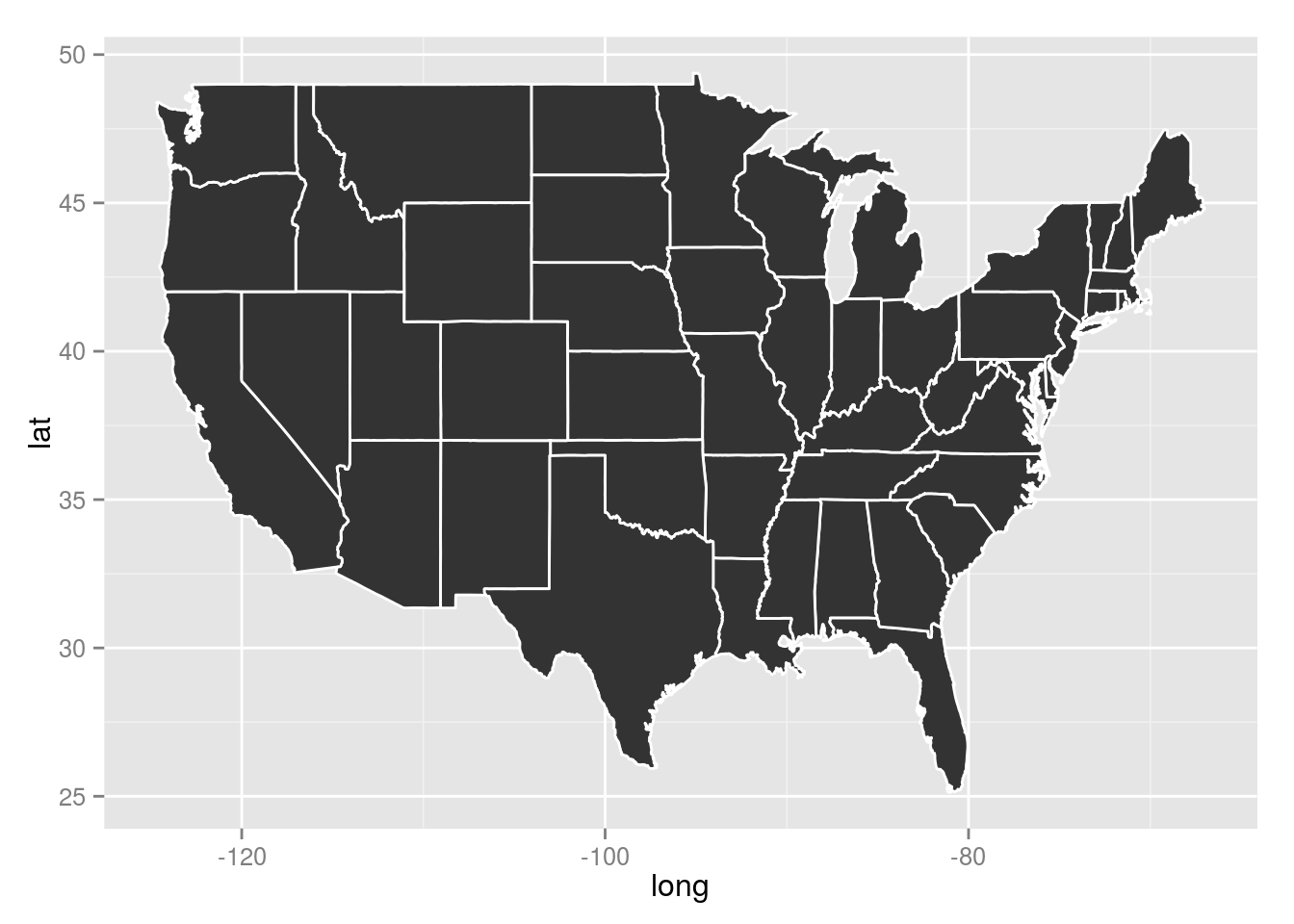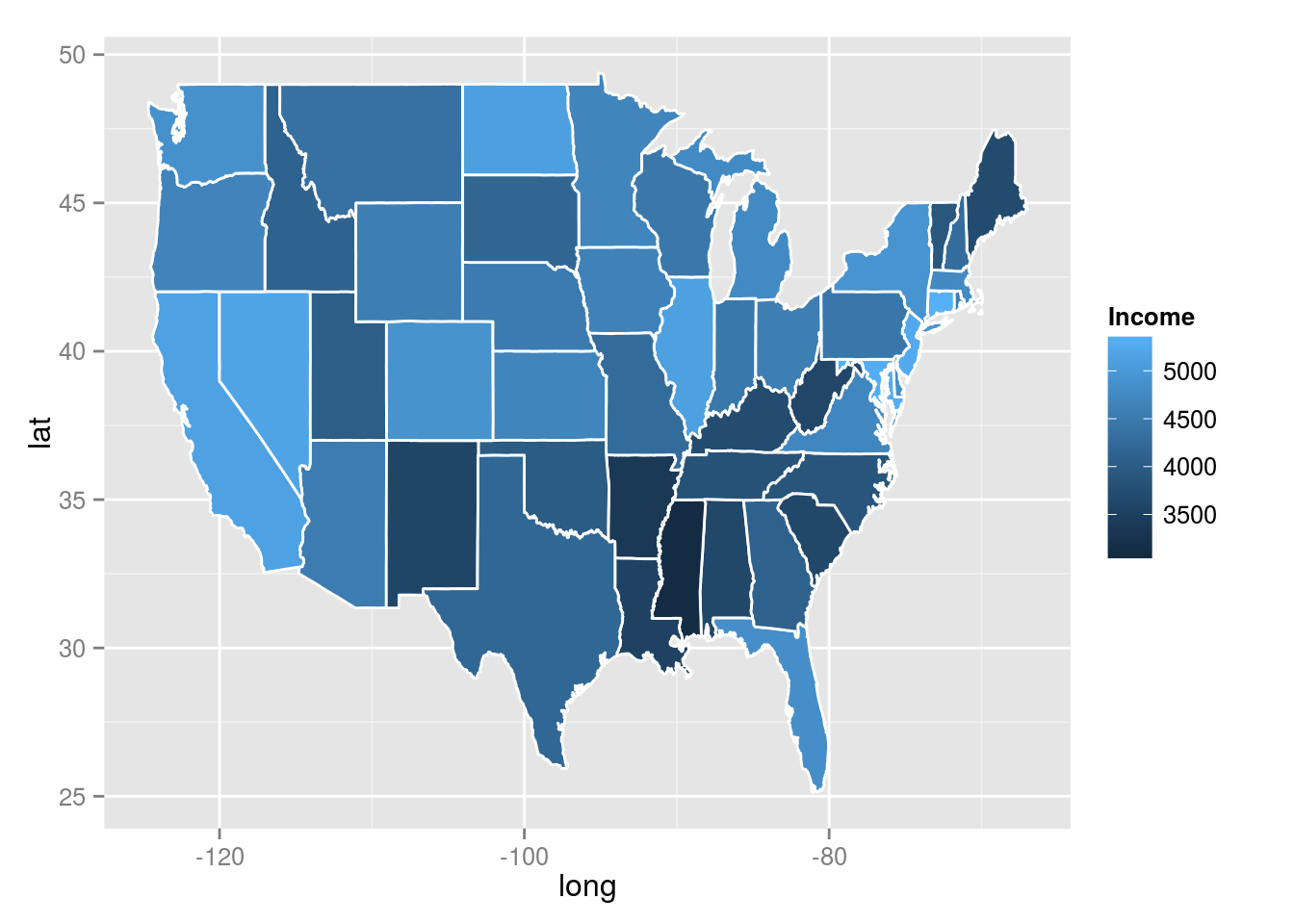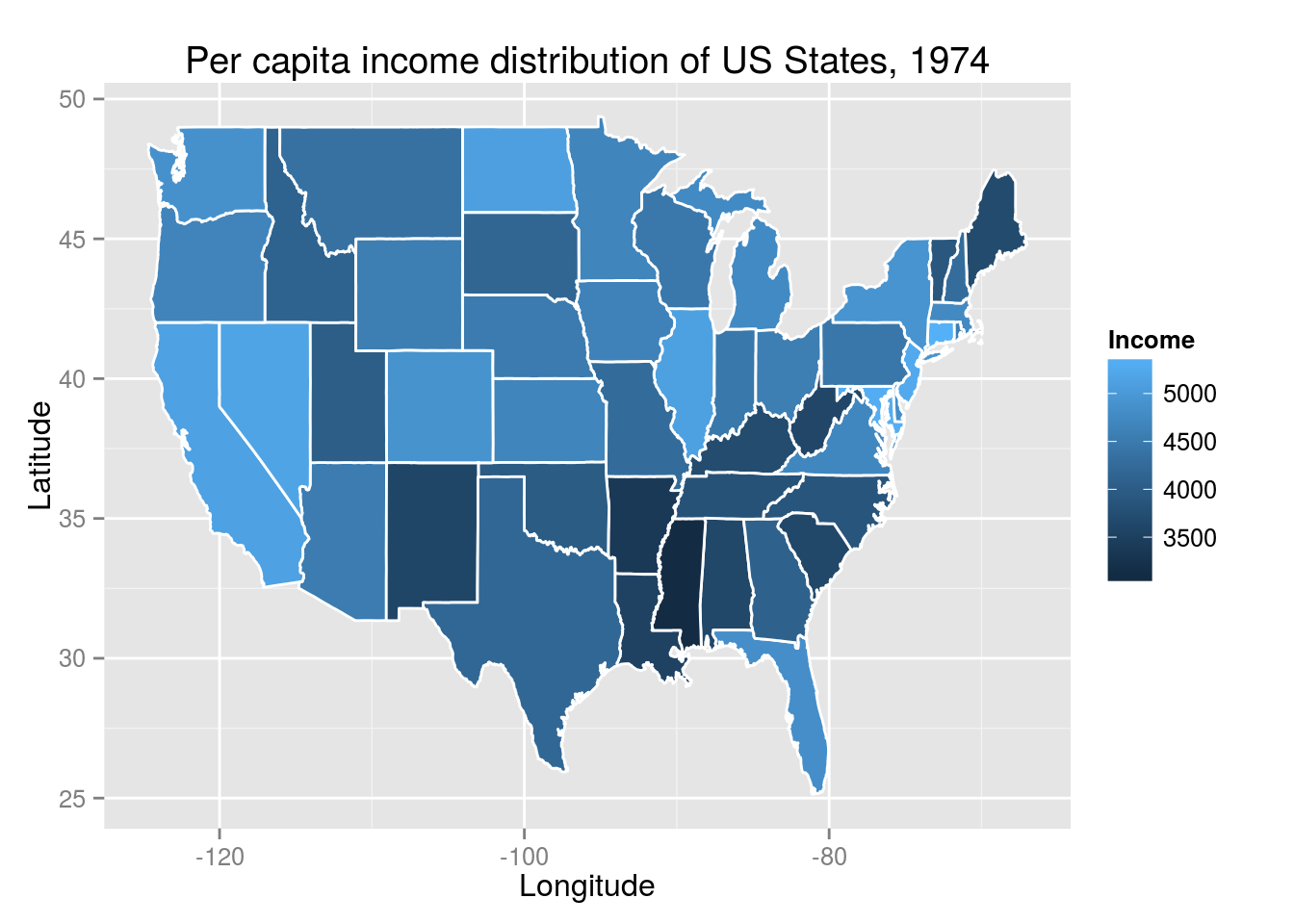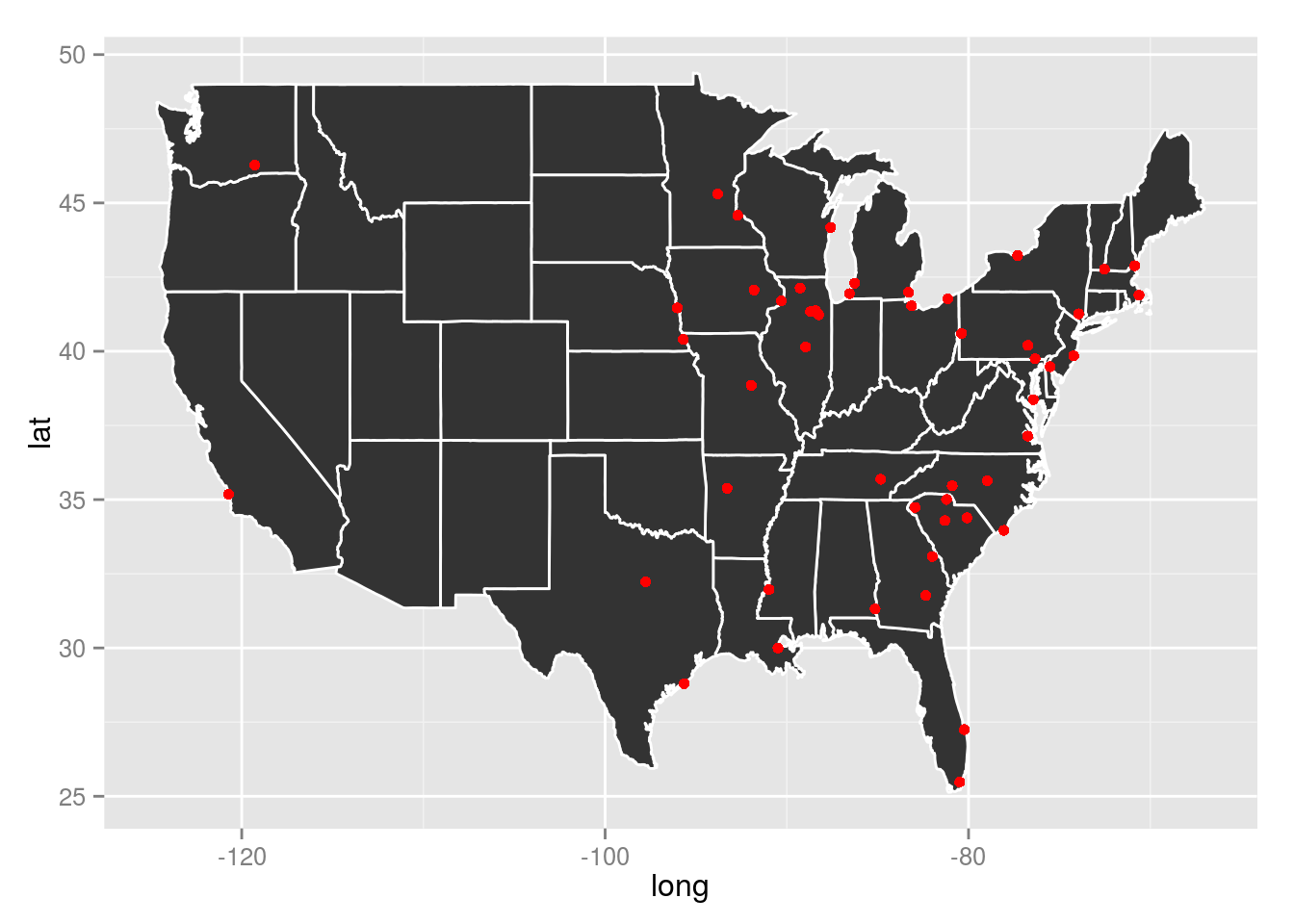Saturday, August 9, 2014

Visualizing Geo-Referenced Data With R

Visualizing Geo-Referenced Data With R

Visualizing Geo-Referenced Data With R

07/01/2014

A key issue in data science is visualization to aid in the telling of stories and the exploration of patterns in data. In this blog post I’m going to look at producing maps that display data with R giving two examples. A large portion of the data of interest to social sciences is actually geo-referenced: election results of electoral districts in a country, worldwide country indicators, locations of conflict hot spots on a map. All these data are screaming for being visualized on maps. I will first outline the required steps for producing maps with geo-referenced data. Then I’ll walk through two examples for two different kinds of geo-referenced data.

Steps

Producing publication quality maps entails a number of steps:

1. Finding maps. While perhaps sounding trivial, it is not so easy to come up with public domain map data that can be used with R. What is required is a data frame that contains the corner points of any borders that should be printed on the map. These borders surround named areas. For example: provincial borders around provinces in a country. This can be produced easily from Shape files that are used with the popular (but proprietary and quite expensive) ArcGIS software package. The maps R package contains a number of maps for the US, Italy, France and New Zealand. It also provides a map of the World.

2. Finding geo-referenced data. If you came this far, then you probably already have some sort of geo-referenced data. Broadly speaking, these can fall into two categories:

• Areas surrounded by borders in the region and identified by name
• Points identified by coordinates in degrees of longitude and latitude
3. Drafting a plot to tell a story.

4. Implement the plot using (e.g.) ggplot2.

Area data example

In these examples I’ll produce a number of different maps using R’s standard data sets. The first map will put key indicators of US States on a map. In the terms of the definition I sketched above, these are area data.

Some of R’s maps are organized in the maps R package. If you don’t have it installed yet, it’s only a quick install.packages("maps") away. After installing it, it is still necessary to load it:

library(maps)

As noted above, the maps package provides a number of maps at varying levels of details. However, these maps are stored in a binary format on disk. In order to turn them into a data frame that can be used for plotting, they need to be converted. This can be achieved by ggplot’s map_data function. Let’s first load the ggplot package, we will need it later for plotting the maps as well.

library(ggplot2)

The map_data function requires only the name of the map that should be converted, for the case at hand this is "state". Conceptionally, the data frame is simply a number of points describing the corners, every corner, of a state. This means a set of points identified by longitude and latitude and and order describing the path that is the border around a state. This is accompanied by a group identifier. The group identifier binds together all those points that describe a single area. In most cases this identical with a state. However, if a state territory also encompasses islands, then these islands form their own respective group each. This explains why 50 states yield the 63 unique groups encoded within this data set. The region identifier finally provides the States’ names. This is the variable where we will later merge the geo-referenced data with. There is also another variable, subregion, that in this map is not used.

us <- map_data("state")
First six entries of the US State map data
long lat group order region subregion
-87.46 30.39 1 1 alabama
-87.48 30.37 1 2 alabama
-87.53 30.37 1 3 alabama
-87.53 30.33 1 4 alabama
-87.57 30.33 1 5 alabama
-87.59 30.33 1 6 alabama

In a next step, let’s look at geo-referenced data. R comes with the state data set that contains a number of data frames with statistical data. The data frame of interest is state.x77. Let’s take a look at it:

data(state)

The data frame state.x77 contains the following variables for each state: . For this map, let’s consider Income:

Min. 1st Qu. Median Mean 3rd Qu. Max.
3100 3990 4520 4440 4810 6320

After having identified a variable, we need to create a data set that merges the shape information from above with the variable of interest. The merge function can be used to this end. However, the map data only covers the continental US and therefore does not contain the states of Alaska and Hawaii. On the other hand, the state data set does not contain data for the District of Columbia. The respective entries from both data sets will therefore be lost in the merge operation.

tmp <- data.frame(region=tolower(rownames(state.x77)),
Income=state.x77[,"Income"])
dta <- merge(x=us, y=tmp)
dta <- dta[order(dta$order),] Let’s start creating the map by setting up the aesthetics. Remember that in ggplot2 aesthetics are used to map data to plot properties. Here, we state that the longitude goes on the x-axis and the latitude on the y-axis. Further, the group identifier separates the individual polygons. p1 <- ggplot(data=dta, aes(x=long, y=lat, group=group)) The ggplot2 geom we need for a map is a polygon. Basically, a polygon is a free shape object that is defined by a path along its borders; exactly what the map data provides. By adding the geom_polygon to the previously set up aesthetics, the map is plotted: p1 + geom_polygon(colour="white")The geom_polygon has two interesting properties: colour to specify the color of the line depicting the border and fill to control the polygon’s fill color. It is fill that we will use to bring our data on the map. In order to add the area data to the plot, the geom_polygon needs to be beefed up with an aesthetics call to fill on it’s own. p1 + geom_polygon(colour="white", aes(fill=Income))We can complete the plot using ggplot2’s usual annotation features: p1 + geom_polygon(colour="white", aes(fill=Income)) + xlab("Longitude") + ylab("Latitude") + ggtitle("Per capita income distribution of US States, 1974")Point data example The second broad type of geo-referenced data are points of interest. Here we will plot the approximate locations of US nuclear power plants. The size of plotting marks will correspond with the power station’s licensed output. First, the data needs to be retrieved. The US Nuclear Regulatory Commission publishes among other things annual reports on all nuclear power plants. Their Appendix A contains some data of all the nuclear power stations in the US and is available as Excel file. There are multiple ways of working with Excel files in R, I find the xlsx package to be quite handy, so we’ll use that to read in the data into R. After reading it in, let’s clean up the data a little as well. library(xlsx) nuclear.raw <- read.xlsx(file = "appa.xls", sheetIndex = 1, startRow = 2, header = TRUE) nuclear <- nuclear.raw[,c(1, 4, 7, 11, 13, 15, 16, 18:23, 25:27)] colnames(nuclear) <- c("Name", "Location", "Type", "Construction", "Operating", "Expiring", "Output", paste0("Capacity", c(12:7,5:3))) Location in this data set is provided by city names and these cities relative locations to larger, better known population centers. We first need to extract the city names and then translate them into Longitude and Latitude. This can be done using the zipcode R package. Let’s extract the city names first. library(stringr) citiesStates <- sapply(strsplit(as.character(nuclear$Location),
split = "(", fixed=TRUE), function(x) return(x))
citiesStates <- t(sapply(strsplit(citiesStates, split=",", fixed=TRUE),
function(x) return(str_trim(x[1:2]))))
colnames(citiesStates) <- c("city", "state")
citiesStates[,"state"] <- toupper(citiesStates[,"state"])
nuclear <- data.frame(nuclear, citiesStates)

This parsing works for most of the names provided by the NRC. Unfortunately, they are occasionally inconsistent. With a little bit more effort, even those cases could be mapped. As this post is more about visualization then regular expressions, let’s settle for the ones where the parsing worked out.

The zipcode package provides a database of zipcodes, city names and their geographic locations. We merge these locations to our nuclear data set, using city and state as key. Unfortunately, not all US nuclear power stations are contained within that database.

data(zipcode, package="zipcode")
nuclear <- merge(nuclear,
zipcode[!duplicated(zipcode[,c("city", "state")]),-1],
all.x=TRUE)
nuclear <- nuclear[!is.na(nuclear[,"longitude"]),]

Now that we’ve built our data set, let’s get about plotting. Basically, we just need to plot points at the correct locations. Let’s start out with an empty map:

p2 <- p1 + geom_polygon(colour="white")
p2We then add a layer of points to that map. The coordinates of the points come from the power stations’ locations. As the map polygons are defined using a group aesthetic, we need to add a constant group variable to the nuclear data as well. It won’t be used, but it needs to be present to appease ggplot.

p2 + geom_point(data=data.frame(nuclear, group=NA),
aes(x=longitude, y=latitude), colour="red")Finally, we can add the Output variable to the aesthetics call to map the power plant’s size to the size of the point on the map. To finalize the plot, we apply the usual beautifications:

p2 + geom_point(data=data.frame(nuclear, group=NA),
aes(x=longitude, y=latitude, size=Output), colour="red") +
xlab("Longitude") + ylab("Latitude") + scale_size_continuous(name="Output (MWh)") +
ggtitle("Location of Commercial Nuclear Power Plants in the US")